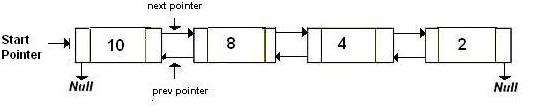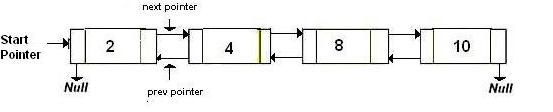GFG App
Open AppBrowser
Continue

# Reverse a Doubly Linked List without swapping nodes

Write a program to reverse the given Doubly Linked List.

See below diagrams for example.

`          (a) Original Doubly Linked List  ``          (b) Reversed Doubly Linked List  `Approach: In the previous post, doubly linked list is being reversed by swapping prev and next pointers for all nodes, changing prev of the head (or start) and then changing the head pointer in the end. In this post, we create a push function that adds the given node at the beginning of the given list. We traverse the original list and one by one pass the current node pointer to the push function. This process will reverse the list. Finally return the new head of this reversed list.

## C++

 `// C++ implementation to reverse` `// a doubly linked list` `#include `   `using` `namespace` `std;`   `// a node of the doubly linked list` `struct` `Node {` `    ``int` `data;` `    ``Node *next, *prev;` `};`   `// function to get a new node` `Node* getNode(``int` `data)` `{` `    ``// allocate space` `    ``Node* new_node = (Node*)``malloc``(``sizeof``(Node));`   `    ``// put in the data` `    ``new_node->data = data;` `    ``new_node->next = new_node->prev = NULL;` `    ``return` `new_node;` `}`   `// function to insert a node at the beginning` `// of the Doubly Linked List` `void` `push(Node** head_ref, Node* new_node)` `{` `    ``// since we are adding at the beginning,` `    ``// prev is always NULL` `    ``new_node->prev = NULL;`   `    ``// link the old list of the new node` `    ``new_node->next = (*head_ref);`   `    ``// change prev of head node to new node` `    ``if` `((*head_ref) != NULL)` `        ``(*head_ref)->prev = new_node;`   `    ``// move the head to point to the new node` `    ``(*head_ref) = new_node;` `}`   `// function to reverse a doubly linked list` `void` `reverseList(Node** head_ref)` `{` `    ``// if list is empty or it contains` `    ``// a single node only` `    ``if` `(!(*head_ref) || !((*head_ref)->next))` `        ``return``;`   `    ``Node* new_head = NULL;` `    ``Node *curr = *head_ref, *next;`   `    ``while` `(curr != NULL) {`   `        ``// get pointer to next node` `        ``next = curr->next;`   `        ``// push 'curr' node at the beginning of the` `        ``// list with starting with 'new_head'` `        ``push(&new_head, curr);`   `        ``// update 'curr'` `        ``curr = next;` `    ``}`   `    ``// update 'head_ref'` `    ``*head_ref = new_head;` `}`   `// Function to print nodes in a` `// given doubly linked list` `void` `printList(Node* head)` `{` `    ``while` `(head != NULL) {` `        ``cout << head->data << ``" "``;` `        ``head = head->next;` `    ``}` `}`   `// Driver program to test above` `int` `main()` `{` `    ``// Start with the empty list` `    ``Node* head = NULL;`   `    ``// Create doubly linked: 10<->8<->4<->2 */` `    ``push(&head, getNode(2));` `    ``push(&head, getNode(4));` `    ``push(&head, getNode(8));` `    ``push(&head, getNode(10));`   `    ``cout << ``"Original list: "``;` `    ``printList(head);`   `    ``// Reverse doubly linked list` `    ``reverseList(&head);`   `    ``cout << ``"\nReversed list: "``;` `    ``printList(head);`   `    ``return` `0;` `}`

## Java

 `// Java implementation to reverse ` `// a doubly linked list ` `class` `GFG` `{`   `// a node of the doubly linked list ` `static` `class` `Node ` `{ ` `    ``int` `data; ` `    ``Node next, prev; ` `}; `   `// function to get a new node ` `static` `Node getNode(``int` `data) ` `{ ` `    ``// allocate space ` `    ``Node new_node = ``new` `Node(); `   `    ``// put in the data ` `    ``new_node.data = data; ` `    ``new_node.next = new_node.prev = ``null``; ` `    ``return` `new_node; ` `} `   `// function to insert a node at the beginning ` `// of the Doubly Linked List ` `static` `Node push(Node head_ref, Node new_node) ` `{ ` `    ``// since we are adding at the beginning, ` `    ``// prev is always null ` `    ``new_node.prev = ``null``; `   `    ``// link the old list of the new node ` `    ``new_node.next = (head_ref); `   `    ``// change prev of head node to new node ` `    ``if` `((head_ref) != ``null``) ` `        ``(head_ref).prev = new_node; `   `    ``// move the head to point to the new node ` `    ``(head_ref) = new_node;` `    `  `    ``return` `head_ref;` `} `   `// function to reverse a doubly linked list ` `static` `Node reverseList(Node head_ref) ` `{ ` `    ``// if list is empty or it contains ` `    ``// a single node only ` `    ``if` `((head_ref) == ``null` `|| ((head_ref).next) == ``null``) ` `        ``return` `null``; `   `    ``Node new_head = ``null``; ` `    ``Node curr = head_ref, next; `   `    ``while` `(curr != ``null``)` `    ``{ `   `        ``// get pointer to next node ` `        ``next = curr.next; `   `        ``// push 'curr' node at the beginning of the ` `        ``// list with starting with 'new_head' ` `        ``new_head = push(new_head, curr); `   `        ``// update 'curr' ` `        ``curr = next; ` `    ``} `   `    ``// update 'head_ref' ` `    ``head_ref = new_head;` `    `  `    ``return` `head_ref;` `} `   `// Function to print nodes in a ` `// given doubly linked list ` `static` `void` `printList(Node head) ` `{ ` `    ``while` `(head != ``null``) ` `    ``{ ` `        ``System.out.print(head.data + ``" "``); ` `        ``head = head.next; ` `    ``} ` `} `   `// Driver program to test above ` `public` `static` `void` `main(String args[])` `{ ` `    ``// Start with the empty list ` `    ``Node head = ``null``; `   `    ``// Create doubly linked: 10< - >8< - >4< - >2 / ` `    ``head = push(head, getNode(``2``)); ` `    ``head = push(head, getNode(``4``)); ` `    ``head = push(head, getNode(``8``)); ` `    ``head = push(head, getNode(``10``)); `   `    ``System.out.print(``"Original list: "``); ` `    ``printList(head); `   `    ``// Reverse doubly linked list ` `    ``head = reverseList(head); `   `    ``System.out.print(``"\nReversed list: "``); ` `    ``printList(head); ` `}` `} `   `// This code is contributed by Arnab Kundu`

## Python3

 `# Python3 implementation to reverse` `# a doubly linked list` `import` `math`   `# a node of the doubly linked list` `class` `Node: ` `    ``def` `__init__(``self``, data): ` `        ``self``.data ``=` `data ` `        ``self``.``next` `=` `None`   `# function to get a new node` `def` `getNode(data):` `    `  `    ``# allocate space` `    ``new_node ``=` `Node(data)`   `    ``# put in the data` `    ``new_node.data ``=` `data` `    ``new_node.``next` `=` `None` `    ``new_node.prev ``=` `None` `    ``return` `new_node`   `# function to insert a node at the beginning` `# of the Doubly Linked List` `def` `push(head_ref, new_node):` `    `  `    ``# since we are adding at the beginning,` `    ``# prev is always None` `    ``new_node.prev ``=` `None`   `    ``# link the old list of the new node` `    ``new_node.``next` `=` `head_ref`   `    ``# change prev of head node to new node` `    ``if` `(head_ref !``=` `None``):` `        ``head_ref.prev ``=` `new_node`   `    ``# move the head to point to the new node` `    ``head_ref ``=` `new_node` `    ``return` `head_ref`   `# function to reverse a doubly linked list` `def` `reverseList(head_ref):` `    `  `    ``# if list is empty or it contains` `    ``# a single node only` `    ``if` `(head_ref ``=``=` `None` `or` `       ``(head_ref).``next` `=``=` `None``):` `        ``return` `None`   `    ``new_head ``=` `None` `    ``curr ``=` `head_ref`   `    ``while` `(curr !``=` `None``):`   `        ``# get pointer to next node` `        ``next` `=` `curr.``next`   `        ``# push 'curr' node at the beginning of the` `        ``# list with starting with 'new_head'` `        ``new_head ``=` `push(new_head, curr)`   `        ``# update 'curr'` `        ``curr ``=` `next` `    `  `    ``# update 'head_ref'` `    ``head_ref ``=` `new_head`   `    ``return` `head_ref`   `# Function to print  nodes in a` `# given doubly linked list` `def` `printList(head):` `    ``while` `(head !``=` `None``) :` `        ``print``(head.data, end ``=` `" "``)` `        ``head ``=` `head.``next`   `# Driver Code` `if` `__name__``=``=``'__main__'``:` `    `  `    ``# Start with the empty list` `    ``head ``=` `None`   `    ``# Create doubly linked: 10<.8<.4<.2 */` `    ``head ``=` `push(head, getNode(``2``)); ` `    ``head ``=` `push(head, getNode(``4``)); ` `    ``head ``=` `push(head, getNode(``8``)); ` `    ``head ``=` `push(head, getNode(``10``));`   `    ``print``(``"Original list: "``, end ``=` `"")` `    ``printList(head)`   `    ``# Reverse doubly linked list` `    ``head ``=` `reverseList(head)`   `    ``print``(``"\nReversed list: "``, end ``=` `"")` `    ``printList(head)`   `# This code is contributed by Srathore`

## C#

 `// C# implementation to reverse ` `// a doubly linked list ` `using` `System;`   `class` `GFG ` `{ `   `// a node of the doubly linked list ` `public` `class` `Node ` `{ ` `    ``public` `int` `data; ` `    ``public` `Node next, prev; ` `}; `   `// function to get a new node ` `static` `Node getNode(``int` `data) ` `{ ` `    ``// allocate space ` `    ``Node new_node = ``new` `Node(); `   `    ``// put in the data ` `    ``new_node.data = data; ` `    ``new_node.next = new_node.prev = ``null``; ` `    ``return` `new_node; ` `} `   `// function to insert a node at the beginning ` `// of the Doubly Linked List ` `static` `Node push(Node head_ref, Node new_node) ` `{ ` `    ``// since we are adding at the beginning, ` `    ``// prev is always null ` `    ``new_node.prev = ``null``; `   `    ``// link the old list of the new node ` `    ``new_node.next = (head_ref); `   `    ``// change prev of head node to new node ` `    ``if` `((head_ref) != ``null``) ` `        ``(head_ref).prev = new_node; `   `    ``// move the head to point to the new node ` `    ``(head_ref) = new_node; ` `    `  `    ``return` `head_ref; ` `} `   `// function to reverse a doubly linked list ` `static` `Node reverseList(Node head_ref) ` `{ ` `    ``// if list is empty or it contains ` `    ``// a single node only ` `    ``if` `((head_ref) == ``null` `|| ((head_ref).next) == ``null``) ` `        ``return` `null``; `   `    ``Node new_head = ``null``; ` `    ``Node curr = head_ref, next; `   `    ``while` `(curr != ``null``) ` `    ``{ `   `        ``// get pointer to next node ` `        ``next = curr.next; `   `        ``// push 'curr' node at the beginning of the ` `        ``// list with starting with 'new_head' ` `        ``new_head = push(new_head, curr); `   `        ``// update 'curr' ` `        ``curr = next; ` `    ``} `   `    ``// update 'head_ref' ` `    ``head_ref = new_head; ` `    `  `    ``return` `head_ref; ` `} `   `// Function to print nodes in a ` `// given doubly linked list ` `static` `void` `printList(Node head) ` `{ ` `    ``while` `(head != ``null``) ` `    ``{ ` `        ``Console.Write(head.data + ``" "``); ` `        ``head = head.next; ` `    ``} ` `} `   `// Driver code` `public` `static` `void` `Main(String []args) ` `{ ` `    ``// Start with the empty list ` `    ``Node head = ``null``; `   `    ``// Create doubly linked: 10< - >8< - >4< - >2 / ` `    ``head = push(head, getNode(2)); ` `    ``head = push(head, getNode(4)); ` `    ``head = push(head, getNode(8)); ` `    ``head = push(head, getNode(10)); `   `    ``Console.Write(``"Original list: "``); ` `    ``printList(head); `   `    ``// Reverse doubly linked list ` `    ``head = reverseList(head); `   `    ``Console.Write(``"\nReversed list: "``); ` `    ``printList(head); ` `} ` `} `   `// This code has been contributed by 29AjayKumar`

## Javascript

 ``

Output:

```Original list: 10 8 4 2
Reversed list: 2 4 8 10```

Time Complexity: O(n).
Auxiliary Space: O(1)

My Personal Notes arrow_drop_up